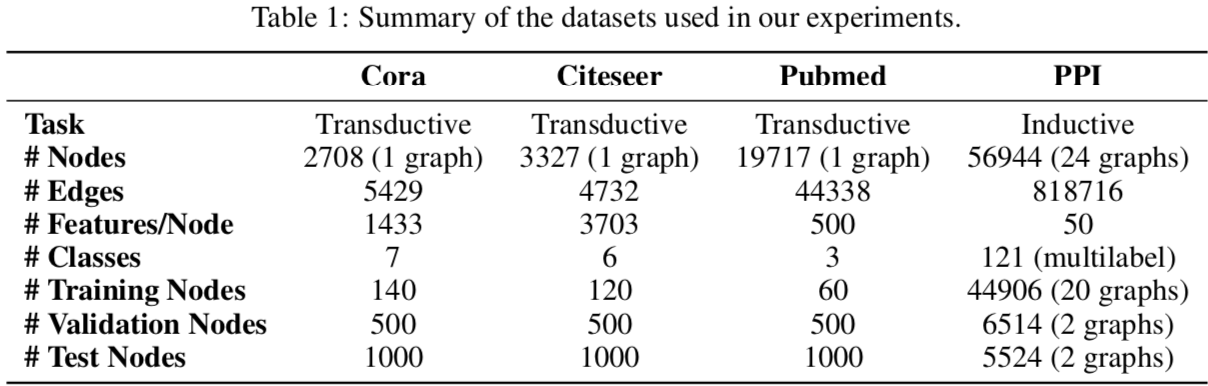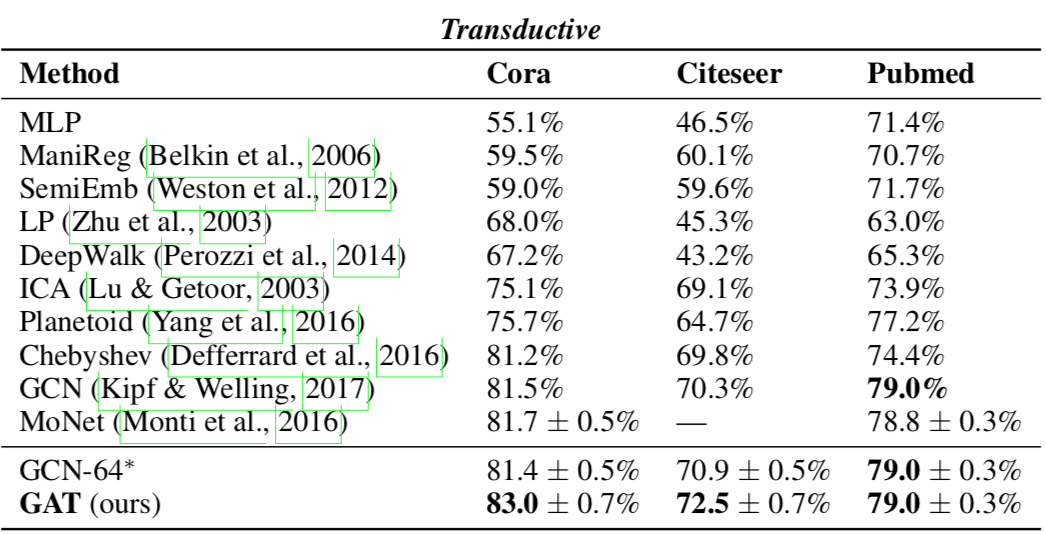# 图注意力网络

GAT 不像之前的网络如 GCN 般在卷积层里固定了每条边的权重（作为卷积核的参数），而是引入自注意力机制用节点的表示计算节点之间的相关性作为边的权重。在尤其是 Inductive Learning 的任务上远超已有方法。

## 模型

• 打分函数：$s_{ij} = \sigma (v^T [h_i; h_j])$，其中 $\sigma$ 作者用的 LeakyReLU
• 权重：$e_{ij} = \text{softmax}(s_{ij})$
• 当前层表示的加权和为下一层的表示（用了 K 个头）：$h_i = W \oplus_{k=1}^K \sigma (\sum\limits_{j \in N_i} e_{ij;k}^T h_j)$

## 实验效果### Transductive Learning### Inductive Learning• GraphSAGE* 是 GraphSAGE 架构下的最好结果，由三层 GraphSAGE-LSTM 组成。
• Const-GAT 设置各边权重相等，按作者说法是为了分别评估注意力机制和其它设计对结果的影响，这样看来最有效的地方在于对多头结果的变换上？